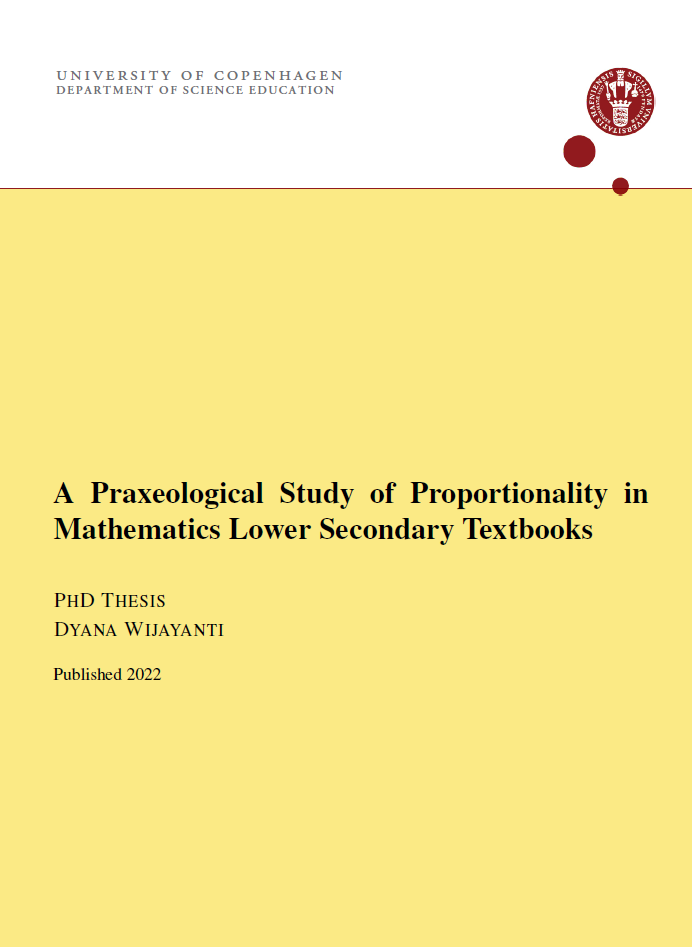5. april 2022

# Nr. 60/2022 A Praxeological Study of Proportionality in Mathematics Lower Secondary Textbooks

Submitted May 2017.

Abstract

Research on the uses and contents of mathematics textbooks has expanded over the past decades, due to the central role textbooks occupy in mathematics teaching worldwide. However, the methodology of analysing the texts themselves often appears underdeveloped or even naïve, especially when it comes to specific mathematical content.

The central idea of this thesis is to deploy the anthropological theory of the didactic, and especially the notion of praxeology, to analyse how textbooks treat three specific and related areas (or more precisely, sectors) of mathematical contents for lower secondary school, namely "proportion and ratio" (in Arithmetic), "similar plane figures" (in Geometry), and "linear functions" (in Algebra). This leads to a new and very precise methodological tool for analysing the practices (types of tasks, techniques) supported by the textbooks through examples, explanations and exercises; it also allows us to analyse how these practices are organised or unified through the theoretical contents, and how the theoretical level may enable the texts to establish explicit links between different sectors.

By constructing praxeological reference models, we obtain a powerful and precise tool to compare the priorities or profiles of different textbooks, their alignment with national examination practices, curricula, etc. We have devoted separate attention to study why certain mathematical links between the three sectors seem to be absent or underdeveloped in textbooks, and explanations are found both in terms of the mathematical notions used (for instance, how "linearity" is defined) and in terms of the historical development of the didactic transposition, as evidenced by textbooks from different periods of time.

Download pdf-version - "A Praxeological Study of Proportionality in Mathematics Lower Secondary Textbooks" [2.8 MB]